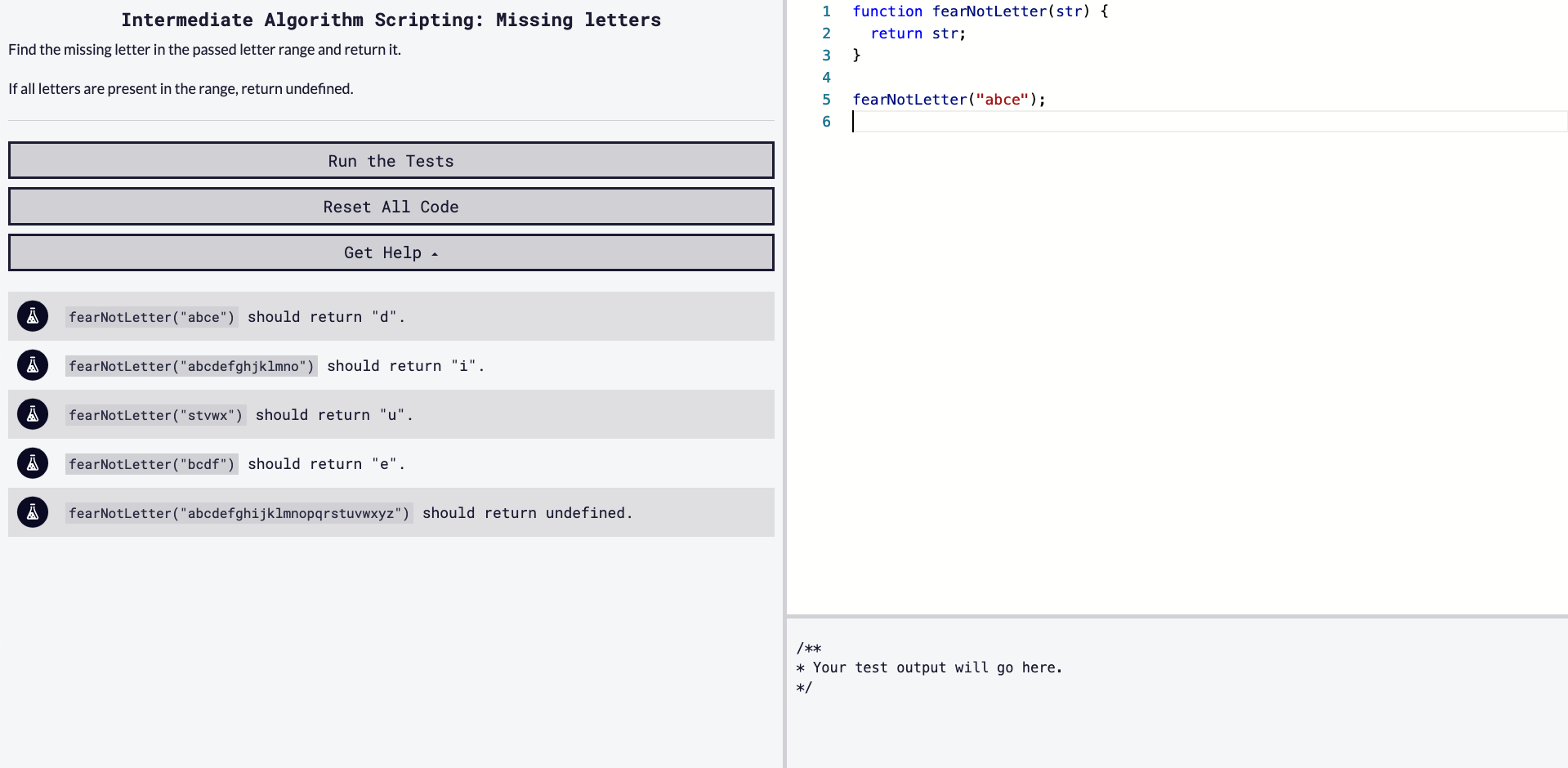## DEV Community

Viren B

Posted on • Originally published at virenb.cc

# Solving "Missing letters" / freeCodeCamp Algorithm ChallengesLet's solve freeCodeCamp's intermediate algorithm scripting challenge, 'Missing letters'.

### Starter Code

``````function fearNotLetter(str) {
return str;
}

fearNotLetter("abce");
``````

### Instructions

Find the missing letter in the passed letter range and return it.

If all letters are present in the range, return undefined.

### Test Cases

``````fearNotLetter("abce") should return "d".
fearNotLetter("abcdefghjklmno") should return "i".
fearNotLetter("stvwx") should return "u".
fearNotLetter("bcdf") should return "e".
fearNotLetter("abcdefghijklmnopqrstuvwxyz") should return undefined.
``````

# Our Approach

We have a short set of instructions for this one. After reading and looking at the test cases,

• We have one input, a string.
• We must return a string, compromised of a single letter, or `undefined`.
• We have to evaluate which letter is missing from the string, `str`. `str` is usually a string of continous (in alphabetical order) lowercased letters.

So, after looking at the test cases, the one which returns `undefined` is a string which contains every letter in the alphabet (in order). The instructions state, 'if all letters are present in the range', return `undefined`.

For this case, I thought we could handle that but doing a `if` statement to check if all the letters were contained. I just made a string of all letters, a-z.

``````if (str == 'abcdefghijklmnopqrstuvwxyz') return undefined;`
``````

You could check the test cases with just this `if` statement and the `undefined` test case should pass. We still have a bit of work to do.

I am thinking we will have to make an array of the alphabet, a-z and also make an array from `str` and compare the two. Let's start off with that.

``````let alphabet = 'abcdefghijklmnopqrstuvwxyz'.split('');
console.log(alphabet)
// Array(26) [ "a", "b", "c", "d", "e", "f", "g", "h", "i", "j", … ]
``````
``````let str = "abcdefghjklmno";
let strArray = str.split('');
console.log(strArray);
// Array(14) [ "a", "b", "c", "d", "e", "f", "g", "h", "j", "k", … ]
``````

So we have two arrays now. We can make a loop to check which one is missing but since `strArray` is just a certain amount of letters, we do not need to loop through the whole alphabet. I'll take the `length` of `strArray` to see how many times we have to execute the loop.

``````let strLen = strArray.length;
``````

Since not all `str` (or `strArray` now) will begin with 'a', we have to check from where to start the comparison between the two arrays. The next variable I am going to create will show us where to begin. We are going to take the `indexOf` `strArray` from `alphabet`. Have a look at the below example -

``````// Test Case: fearNotLetter("stvwx") should return "u".
str = "stvwx";
let strArray = str.split('');
let idx = alphabet.indexOf(strArray);
let strLen = strArray.length;

// strArray = ['s', 't', 'v', 'w', 'x'];
// idx = 18; // 18 is the index of 's' in the alphabet
// strLen = 5;

``````

So I think we have all the information we need to make the comparison. We have an alphabet array, a string array (to compare), the index to start the comparison at, and length to see how many times we need to run the loop to check.

To do this, I would like to do is run `splice()` on `alphabet` in order to get the exact part of the alphabet to compare.

``````let splicedAlphabet = alphabet.splice(idx, strLen);
``````

So, we are exacting (from `alphabet`), the starting `idx`. For example

``````// Test Case: fearNotLetter("abce") should return "d".
alphabet = [ "a", "b", "c", "d", "e", "f", "g", "h", "i", "j", … ]
strArray = [ "a", "b", "c", "e" ]
let strLen = strArray.length; // 4
let idx = alphabet.indexOf(strArray) // Checking where 'a' is, 0 index
let splicedAlphabet = alphabet.splice(idx, strLen);

// splicedAlphabet is taking (0, 4) // ['a', 'b', 'c', 'd']
``````

So, with `splicedAlphabet`, we can check this against `strArray` to find the difference (or missing letter).

To execute the comparsion, I will use a `for` loop. Will run it on `splicedAlphabet`'s length.

``````for (let i = 0; i < splicedAlphabet.length; i++) {
if (!strArray.includes(splicedAlphabet[i])) return splicedAlphabet[i];
}
``````

So, we are looking to see which letter is missing. We run an `if` statement within the `for` loop. If `strArray` doesn't include `splicedAlphabet[i]`, that would be the missing letter, so we return it.

Array.includes() (MDN)

# Our Solution

``````function fearNotLetter(str) {
if (str == 'abcdefghijklmnopqrstuvwxyz') return undefined;

let alphabet = 'abcdefghijklmnopqrstuvwxyz'.split('');
let strArray = str.split('');
let idx = alphabet.indexOf(strArray);
let strLen = strArray.length;
let splicedAlphabet = alphabet.splice(idx, strLen);

for (let i = 0; i < splicedAlphabet.length; i++) {
if (!strArray.includes(splicedAlphabet[i]))
return splicedAlphabet[i]
}
}

fearNotLetter("abce");
``````

'Missing letters' Challenge on fCC

freeCodeCamp

Donate to FCC!

Solution on my GitHubfunction fearNotLetter(str) {
let dummy = 'abcdefghijklmnopqrstuvwxyz'
let x = dummy.slice(dummy.indexOf(str))
let s='';

for(let j=0;j<x.length;j++){
if(str.slice(-1) == x.charAt(j)){
s = s.concat(x.charAt(j))
break;
}
else {
s = s.concat(x.charAt(j))
}
}

return s[[...str].findIndex((el, index) => el !== s[index])]
}

fearNotLetter("abce");

DEV Community